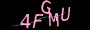×

# 谈谈二进制的乘法和除法A由左到右左移进入D。每次移位后比较D和B的值

A=1100 左移进入D,A=100

D=1 B=110;D<B;

C=0

A=100 左移进入D,A=00

D=11 B=110 D≥B成立;

11-110=0

D=0

C=01

A=00左移进入D,A=0

D=0 B=110 D<B

C=010

A=0左移进入D,A完毕

D=0 B=110;D<B;

https://www.jiangweishan.com/article/codeYuanmaList2.html

### 网友评论文明上网理性发言已有0人参与